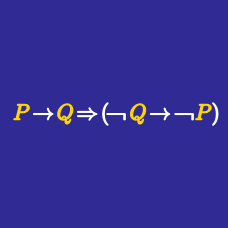Logic

# Basic Logic Gates Warmup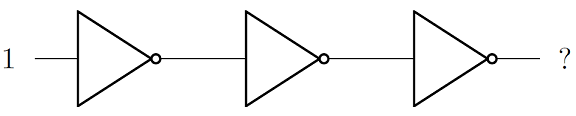The gates above are "inverters" or "NOT gates", that switch high voltage (1) to low voltage (0) or vice versa. What is the output?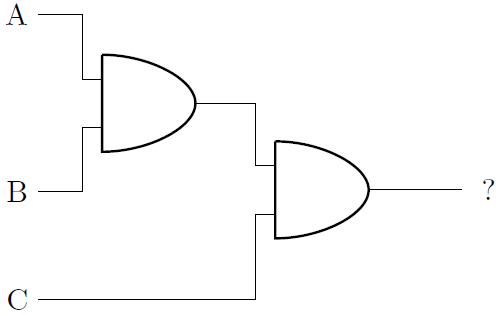The AND gates in the diagram will output a 1 if both inputs are also 1.

If A = 1, B = 1, and C = 0, what will the final output be?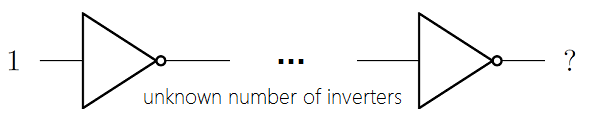Only picking out of the choices listed, how many inverters (or NOT gates) are needed so that the output of the diagram is 1?How many different ordered triples are there (A,B,C) such that the output of these AND logic gates is 0?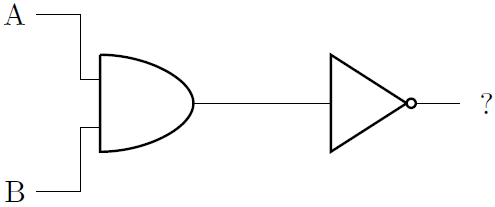What will the output be if A = 1 and B = 0?

×